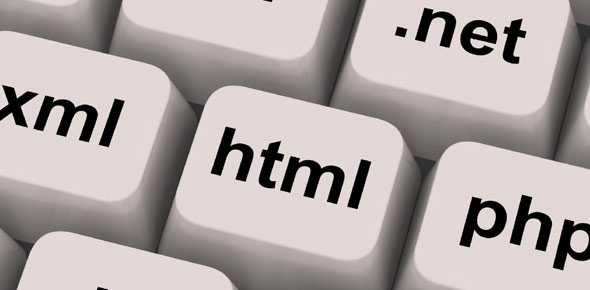# C Language Quiz @ Aptech Dha

35 Questions | Attempts: 159
ShareSettings• 1.
Do while loop tests the condition at the End of the Loop.
• A.

True

• B.

False

• 2.
The Symbol && is read as ____________________.
• A.

AND

• B.

OR

• 3.
What type of errors are checked during compilation
• A.

Logical errors

• B.

Divide by zero error

• C.

Run - time errors

• D.

Syntax errors

• 4.
The purpose of main function is
• A.

To stop program execution

• B.

To stop algorithm

• C.

To start algorithm

• D.

To start program execution

• 5.
What is the valid identifier in the following
• A.

1fdasgf

• B.

@hgd12

• C.

Fahs%*

• D.

Q1234

• 6.
What is range of char data value?
• A.

-64 to 64

• B.

-128 to 127

• C.

0 to 255

• D.

-127 to 128

• 7.
The minimum number of temporary variable needed to swap the contents of two variable is
• A.

3

• B.

1

• C.

0

• D.

2

• 8.
In the expression b=6.6/a+(2*a+(3*c)/a*d)/(2/n); which operation will be performed first?
• A.

2*a

• B.

2/n

• C.

3*c

• D.

6.6/a

• 9.
Which of the following is an incorrect variable name.
• A.

Else

• B.

Name

• C.

string

• D.

Age

• 10.
Variables are initialized in C, using
• A.

: =

• B.

>

• C.

=

• D.

==

• 11.
In the following, which is bitwise operator?
• A.

>

• B.

*

• C.

|

• D.

• 12.
What is output of the following program? main() {int a; float b; a=1/2; b=1.0/2.0; printf(“ a=%d b=%f”,a,b); }
• A.

A=0.0 b=0. 5

• B.

A=0 b=0. 5

• C.

A=0.0 b= 5

• D.

A=0.5 b=0. 5

• 13.
Representing various steps in a flow diagram is called as
• A.

Diagram

• B.

Flow chart

• C.

Program

• D.

Paint

• 14.
What are the smallest individual units in a program
• A.

Structures

• B.

Functions

• C.

record

• D.

Tokens

• 15.
The operator ++ is called as operator
• A.

Special increment

• B.

Increment

• C.

• D.

Decrement

• 16.
Which of the following is not a relational operator
• A.

• B.

&&

• C.

!=

• D.

>

• 17.
main ( ) { int m,y; m = 5; y = ++m; printf(”%d %d”,m,y);} consider the above code and find the output
• A.

5,5

• B.

5,6

• C.

6,6

• D.

6,5

• 18.
Int C; C=25/2; What is the value of C
• A.

0.5

• B.

12.500000

• C.

12.000000

• D.

12

• 19.
Which of the following cannot be used as an identifier.
• A.

Alphabet followed by digit

• B.

Alphabet

• C.

Keywords

• D.

Library function name

• 20.
Size of (double) returns
• A.

4

• B.

8

• C.

10

• D.

2

• 21.
The ___________________ loop executes at least once.
• A.

Do while

• B.

While

• 22.
An ___________________ is a collection of variables having same data type
• A.

Array

• B.

Operator

• C.

Function

• 23.
What will be the output of the following program? #include int main() { int a = 1, b = 2, c = 3; printf("The value of a is %d", a); a+=3; printf(" The value of a is %d", a); return 0; }
• A.

The value of a is 2 The value of a is 2

• B.

The value of a is1 The value of a is 3

• C.

The value of a is 1 The value of a is 4

• D.

Compiler error

• 24.
What  is the output of the below program? What is value of C. { a=10; b=12; c=a+b*2; }
• A.

44

• B.

22

• C.

240

• D.

Compiler Error

• 25.
What will be the output of the following program? #include<stdio.h> int main() { int arr= {1, 2, 3, 4}; printf("%d\n",arr ); }
• A.

3

• B.

Compiler Error

• C.

Runtime Error

• D.

4

## Related TopicsBack to top
×

Wait!
Here's an interesting quiz for you.